Importance: High ✭✭✭
 Author(s): Erdos, Paul Hajnal, Andras
 Subject: Graph Theory » Extremal Graph Theory
 Keywords: induced subgraph
 Posted by: mdevos on: March 18th, 2007
Conjecture   For every fixed graph, there exists a constant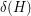, so that every graphwithout an induced subgraph isomorphic tocontains either a clique or an independent set of size.

There are numerous interesting classes of graphs which are based upon forbidding one or more induced subgraphs. For instance: chordal graphs, split graphs, and claw-free graphs. Numerous other natural classes of graphs have been proved to have such characterizations, most famously perfect graphs, but also line graphs and comparability graphs. All of these classes are very well structured (far from random) and their members all either have large cliques or independent sets. On the flip side of this are random graphs. It is well known that a random graph onvertices has both clique and independence number highly concentrated around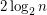. The Erdos-Hajnal conjecture suggests a fundamental separation between these two worlds in terms of independence/clique sizes.

Erdös and Hajnal proved that this conjecture is true for the recursive class of graphsdefined as follows. The one vertex graph is in, and ifandlie in, then the disjoint union ofandlies in, as does the graph obtained from the disjoint union by adding an edge between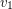and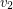for every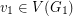and. More generally, Alon, Pach, and Solymosi proved that ifis a graph with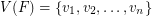for which the Erdös-Hajnal conjecture holds, and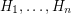are graphs for which the Erdos-Hajnal conjecture holds, then the graph obtained fromby blowing up each vertexwith a copy of(more precisely, starting from the disjoint union of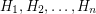, we add all possible edges between the vertices ofand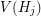if) also satisfies the Erdos-Hajnal conjecture.

The Erdös-Hajnal property is known to hold for a number of small graphs (and using the above result this may be easily bootstrapped). For instance, the conjecture is known to hold whenis a path of three edges, and recently M. Chudnovsky and S. Safra have announced a proof whenis a bull (a triangle plus two pendant edges). However, our knowledge here is still quite limited. In particular, Lovasz has suggested the following very special case which remains open.

Question   Is the Erdös-Hajnal conjecture true when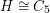?

## Bibliography

[APS] N. Alon, J. Pach, and J. Solymosi, Ramsey-type theorems with forbidden subgraphs, Combinatorica 21 (2001), 155-170.

[EH] P. Erdös and A. Hajnal, Ramsey-type theorems, Discrete Appl. Math. 25 (1989), 37-52 MathSciNet

* indicates original appearance(s) of problem.